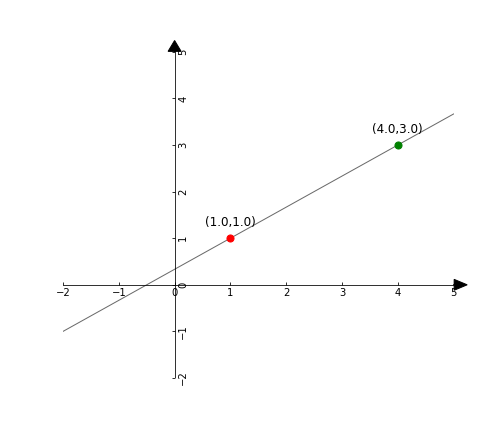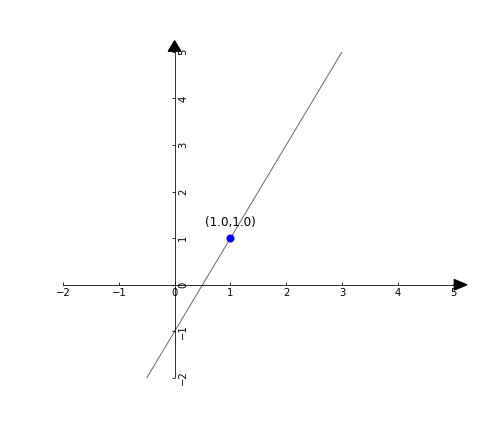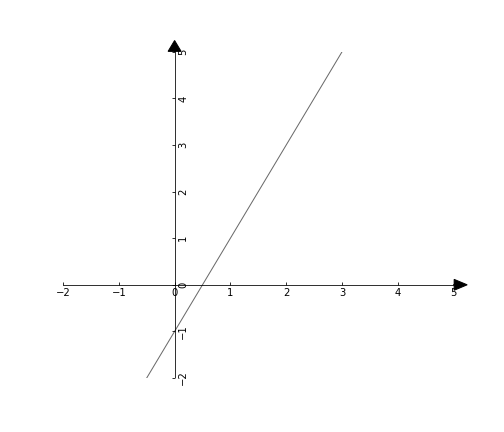# Python: Draw a line in a Cartesian coordinate system

For now, there are three ways to draw a line with my pyGeom package.

To install the package:

# Step 1: Install the package using pip
\$ pip install pyGeom2D

Then

# You will need to import the Axes, Point, and Line classes
from pygeom import Axes, Point, Line

### Method 1: Using two points

# Create the cartesian axis
axes = Axes(xlim=(-2,5), ylim=(-2,5), figsize=(7,6))

# Line between two points
p1 = Point(1, 1, color='red')
p2 = Point(4, 3, color='green')

l = Line(p1=p1, p2=p2)

axes.draw()### Method 2: Using a point and a slope

Remember, when your line is defined using two points, say

$$P1 = (x_1, y_1)$$ and

$$P2 = (x_2, y_2)$$, , you can compute the slope of your line by:

$$slope = \frac{y_2 – y_1}{x_2 – x_1} \quad \text{“rise over run”}$$

But if you know the slope of your line, you only need one point to draw the line.

# Create the cartesian axis
axes = Axes(xlim=(-2,5), ylim=(-2,5), figsize=(7,6))

# Point and slope
p = Point(1, 1, color='blue')
slope = 2.0

l = Line(p=p, slope=slope)

axes.draw()### Method 3: Using a slope and an intercept

In this form, you are basically providing an equation for your line. The slope-intercept form is

$$y = mx + b$$

where m is the slope and b is the intercept (the point at which the line intercepts the y-axis).

So if your line equation is

$$y = 2x – 1$$

# Create the cartesian axis
axes = Axes(xlim=(-2,5), ylim=(-2,5), figsize=(7,6))

l = Line(slope=2.0, intercept=-1.0)

axes.draw()